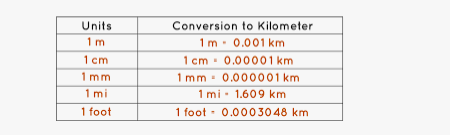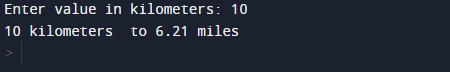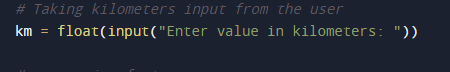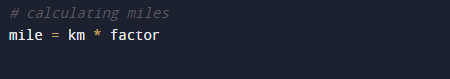# Python Program to Convert Kilometers to Miles

In this tutorial you will learn about the Python Program to Convert Kilometers to Miles and its application with practical example.

In this tutorial, we will learn to create a Python Program to Convert Kilometres to Miles  using python Programming.

## Prerequisites

Before starting with this tutorial we assume that you are best aware of the following Python programming topics:

• Python Operator.
• Basic Input and Output
• Basic Python  programming.
• Python if-else statements.
• Python Loop.

## What is kilometre?

A Unit of measuring Length which is equal to 1,000 meters. So we can say that                          => ‘1 Kilometre = 1,000 Meters’.The Algorithm is as follow :

1. First of all ask user to enter value in kilometre which will be assign to variable ‘km’.
2. Factor of kilometre to mile is approx 0.621371 miles.
3. So,to get the value in mile multiply the  inputted value with factor with km*factor .
4. Print  the required result  in miles.
5. Exit the program.

## Python Program to Convert Kilometres to Miles

In our Program below we will create a program that will Convert Kilometre to Miles.First we would declared and initialized the required variables. Next, we would prompt user to input value in kilometre.Later we will covert entered value into meter.

## OutputIn our program, we have first declared and initialized a set variables required in the program.

• km =taking year value from user.
• mile=hold converted value of kilometres.

First of all  we declare variable and take a value from user and assign in ‘km’ and convert it into miles .After taking values from user we will add conversion factor ‘ factor = 0.621371‘ to variable Factor.Another Mile’s unit of length is Equal to ‘1760 yards’. Since 1 kilometre is equal to 0.621371 miles, we can get the value of miles by simply multiplying kilometres with the factor (km* 0.621371).And Finally we have learnt to convert kilometres to miles and display it.In this tutorial we have learn about the Python Program to Convert Kilometers to Miles and its application with practical example. I hope you will like this tutorial.